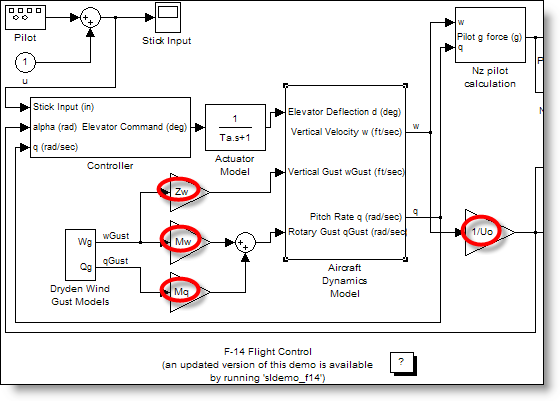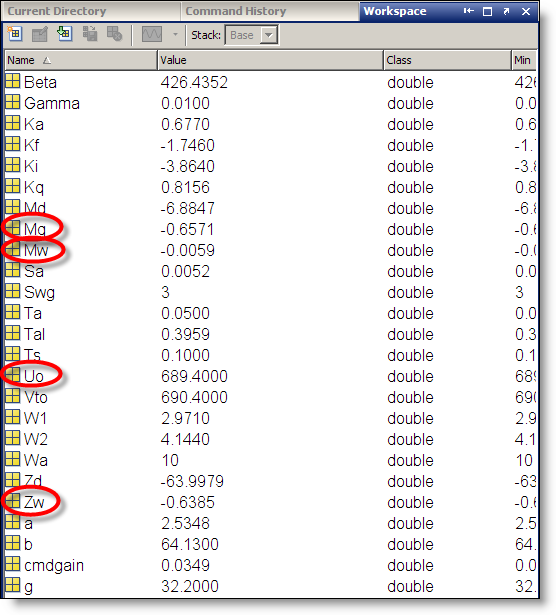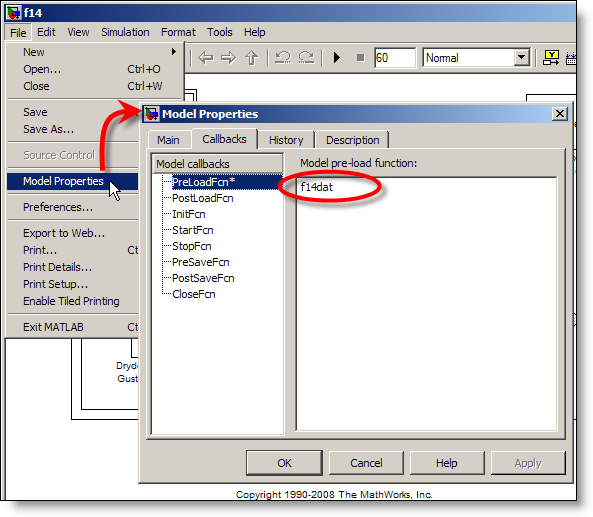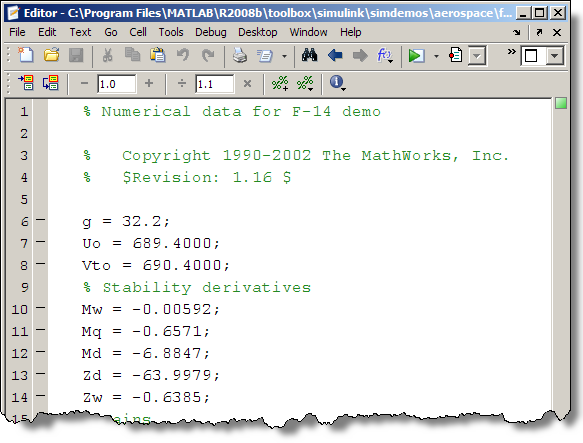# Initializing Parameters

Simulink models often use parameters from the MATLAB base workspace.  However, Simulink does not save the MATLAB base workspace with the model.  This leads me to the following question: How do you initialize the workspace for your simulation?

Most Simulink Demos initialize the base workspace before they are loaded.  Let’s take a look at f14.mdl:In the F14 model, you see gain blocks with parameters like Zw, Mw, Mq and 1/Uo.  Simulink evaluates those MATLAB expressions in the base workspace during initialization of the simulation.You can register model callbacks that run when you perform different actions on the model.  In this example, f14.mdl has a PreLoadFcn that runs a script to initializes the workspace.  You can see the model callbacks by opening the Model Properties from the File menu.The model pre-load function executes before the model is actually loaded.  If you only need to initialize a few variables for your model, you can enter the MATLAB expressions directly in the pre-load function callback.  The F14 model calls the M-file script f14dat.m.If I am tweaking variables in my model, I like to change them directly in the workspace browser, or at the command line.  The pre-load function is a good place for initializing variables because it only runs the first time the model is loaded.  This allows you to change the variables for the current session without modifying the initialization code.

Other techniques for initialization?

How do you go from the blinking MATLAB cursor to a fully initialized environment?  Leave a comment here and share your model initialization tips.

|